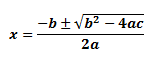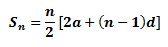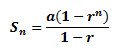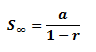# Algebra Formula: Definition, Formulas and Examples

0
435

## Special Algebra Expansions

#### The roots for this quadratic equation will be:## Arithmetic Progression

#### The sum of the first n terms of the arithmetic progression is:## Geometric Progression

#### The sum of the first n terms, Sn is:#### If -1 < r < 1, the sum to infinity, S∞ is:READ HERE  Velocity formula with Relevant Examples